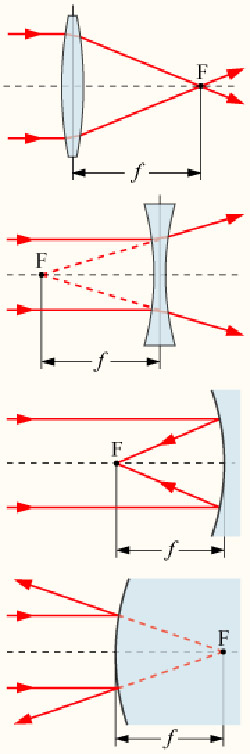A# focal lengthThe focal length is the distance between a lens or a mirror and its focal point. In the case of a converging lens or mirror, it is where the light is concentrated. For diverging systems the focus is virtual in that it lies at the vertex of the rays is they were extend away from the direction of propagation.

The focal length of a compound optical system, given as if it were a single optical element, is called the equivalent focal length. The focal length at which an optical system seems to be working in a given situation is known as the effective focal length. For example, if an eyepiece projects an image onto a film, the image is magnified as if it were made by a telescope of several times the telescope's equivalent focal length. The back focal length is the distance between the last surface of a compound optical system and the focal plane of the system and may be quite different from the actual focal length.